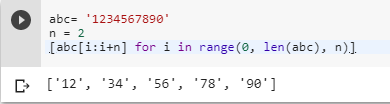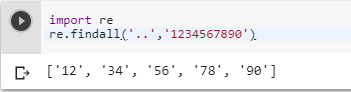# Split string every nth character?

1 view
in Python

Is it possible to split a python string every nth character?

For example, suppose I have a string containing the following:

'1234567890'

How can I get it to look like this:

['12','34','56','78','90']

by (107k points)

There are many ways by which you can split string’s every nth character some of the import methods are as follows:-

The first method you can use to solve this problem is by using the len() function.

abc= '1234567890'

n = 2

[abc[i:i+n] for i in range(0, len(abc), n)]The second way of solving this problem is by using the regular expression module and its findall() function below is the code that shows how we can use the regular expressions to split string by nth char:-

import re

re.findall('..','1234567890')